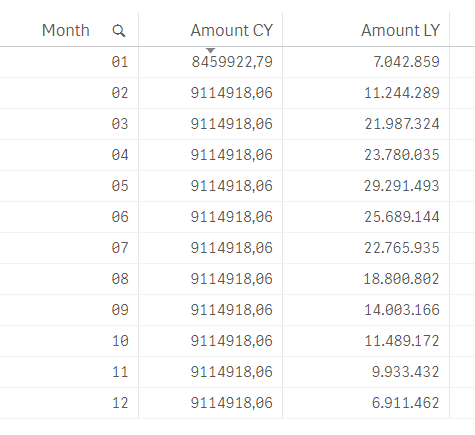New to QlikView

Discussion board where members can get started with QlikView.

Announcements
QlikWorld 2020: Join us May 11 - 14, 2020 in Phoenix, AZ. Register early and save \$400. Learn More
HighlightedPartner

Accumulative sum till current month

Hi all,

I'm having some trouble figuring the following out.

I have a accumulation per month of sum(amount) for the current year and previous year. But my line chart for the current year should stop at the current month.

I've used the following formula for the calculation of the accumulation:

rangesum(above(Sum({<Year={\$(=max(Year))}>}Amount),0,rowno()))

The calculation works just fine. See the results in the table below:As a result my linechart looks like this:But my client wants the Amount CY line to stop at month 02.

Is there a way to get this done?

Thank you in advance!

1 Solution

Accepted Solutions
Esteemed Contributor II

Re: Accumulative sum till current month

=if(Month <= Month(today()) , rangesum(above(Sum({<Year={\$(=max(Year))}>}Amount),0,rowno())))

3 Replies
Esteemed Contributor II

Re: Accumulative sum till current month

=if(Month <= Month(today()) , rangesum(above(Sum({<Year={\$(=max(Year))}>}Amount),0,rowno())))

Esteemed Contributor II

Re: Accumulative sum till current month

or give this a try

=rangesum(above(Sum({<Year={\$(=max(Year))} , Month = {"<=\$(=Month(Today()))"}>}Amount),0,rowno())))Partner

Re: Accumulative sum till current month

Thank you so much Vineeth!

I tried several things but I must have missed this one.